Rational and Irrational Values Terms of Use    Contact Person: Donna RobertsDirections:
Decide whether the following statements are TRUE or FALSE and check your answer at the right. Remember, TRUE means "true under any and all conditions".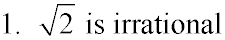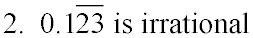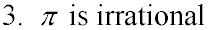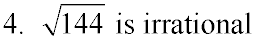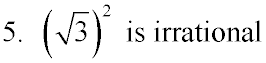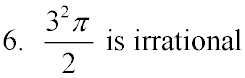Choose:
 1 TRUE FALSE
 2 TRUE FALSE
 3 TRUE FALSE
 4 TRUE FALSE
 5 TRUE FALSE
 6 TRUE FALSE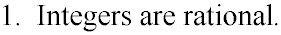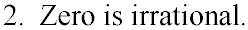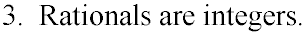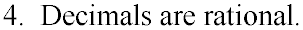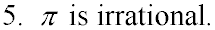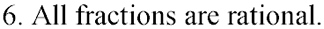Choose:
 1 TRUE FALSE
 2 TRUE FALSE
 3 TRUE FALSE
 4 TRUE FALSE
 5 TRUE FALSE
 6 TRUE FALSE

Directions: Answer these questions pertaining to working with rational and irrational values.

1.
Which choice is not irrational?Choose: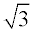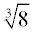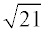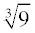2.
Which choice is not rational?Choose: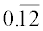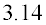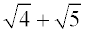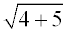3.
Which of the following lengths and widths represent a rectangle whose diagonal is rational?Choose:
 length = 5, width = 4 length = 4, width = 3 length = 3, width = 2 length = 2, width = 1

4.
Which choice is real, rational, and integer?Choose: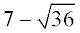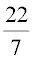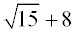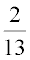5.
Between which two numbers does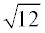lie?Choose:
 11 and 12 6 and 7 4 and 5 3 and 4

6.
One leg of a right triangle measures 8 units and the hypotenuse measures 12 units. The perimeter of the triangle is irrational.Choose:
 TRUE FALSE